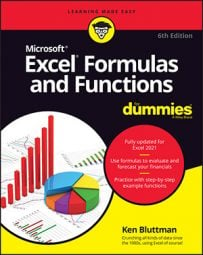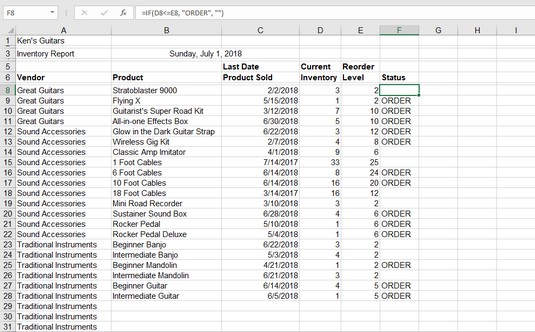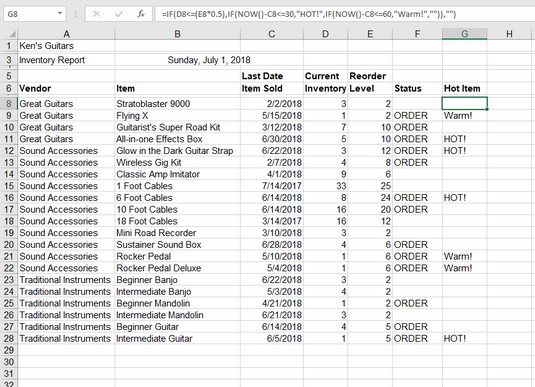##### Excel Formulas & Functions For DummiesExcel’s IF function is like the Swiss Army knife of Excel functions. Really, it is used in many situations. Often, you can use Excel’s IF function with other functions. IF, structurally, is easy to understand. The Excel IF function takes three arguments:
• A test that gives a true or false answer. For example, the test `"is the value in cell A5 equal to the value in cell A8"` can have only one of two possible answers, yes or no. In computer talk, that's true or false. This is not a calculation, mind you, but a comparison.
• The data to be returned by the IF function if the test is true.
• The data to be returned by the IF function if the test is false.
Sounds easy enough. Here are some examples:
 Function Comment `=IF(D10>D20, D10, D20)` If the value in D10 is greater than the value in D20, the value in D10 is returned because the test is true. If the value in D10 is not greater than — that is, smaller or equal to — the value in D20, the value in D20 is returned. If the values in D10 and D20 are equal, the test returns false, and the value in D20 is returned. `=IF(D10>D20, "Good news!", "Bad news!")` If the value in D10 is greater than the value in D20, the text “Good News!” is returned. Otherwise, “Bad News!” is returned. `=IF(D10>D20, "", "Bad news!")` If the value in D10 is greater than the value in D20, nothing is returned. Otherwise, “Bad News!” is returned. Note that the second argument is a pair of empty quotes. `=IF(D10>D20, "Good news!", "")` If the value in D10 is greater than the value in D20, “Good News!” is returned. Otherwise, nothing is returned. Note that the third argument is empty quotes.

An important aspect to note about using IF: letting the second or third argument return nothing. An empty string is returned, and the best way to do this is to place two double quote marks together with nothing in the middle. The result is that the cell containing the IF function remains blank.

IF, therefore, lets you set up two results to return: one for when the test is true and another for when the test is false. Each result can be a number, some text, a function or formula, or even blank.

As you see in the preceding example, a common use of IF is to see how two values compare and return either one value or the other, depending on how you set up the test in the first argument.

IF is often used as a validation check to prevent errors. Suppose that you have a financial worksheet that uses a variable percentage in its calculations. The user must enter this percentage each day, but it must never be greater than 10 percent. To prevent the chance of errors, you could use the IF function to display an error message in the adjacent cell if you mistakenly enter a value outside the permitted range. Assuming that the percentage is entered in cell A3, here's the required IF function:

`=IF(A3>.1, "ERROR: the % in A3 IS TOO LARGE", "")`
The following image shows how IF can be put to good use in a business application. A fictitious store shop — Ken's Guitars (kinda snappy, don’t you think?) — keeps tabs on inventory in an Excel worksheet.Keeping an eye on inventory at the guitar shop.

Column D shows the inventory levels, and column E shows the reorder levels. It works this way: When a product's inventory level is the same or less than the reorder level, it is time to order more of the product. The cells in column F contain a formula.

The Excel formula in cell F8 is `=IF(D8<=E8,"ORDER","")`. It says that if the number of Stratoblaster 9000 guitars in stock is the same or less than the reorder level, return `Order`. If the number in stock is greater than the reorder level, return nothing. Nothing is returned because three are in stock and the reorder level is two. In the next row, the number of Flying Xs is equal to the reorder level; therefore, cell F9 displays `Order`.

Using Excel’s IF function is easy. Follow these steps:

1. Enter two values in a worksheet.These values should have some meaning to you, such as the inventory levels example shown above.
2. Click the cell where you want the result to appear.
3. Type =IF( to start the function.
4. Decide what test you want to perform.You can see whether the two values are equal; whether one is larger than the other; whether subtracting one from the other is greater than, equal to, or less than 0; and so on. For example, to determine whether the first value equals the second value, click the first cell (or enter its address), enter an equal sign (=), and then click the second cell (or enter its address).
5. Type a comma (,).
6. Enter the result that should appear if the test is true.

For example, enter “The values are equal”. Text must be enclosed in quotes.

7. Type a comma (,).
8. Enter the result that should appear if the test is false.For example, enter “The values are not equal”.
9. Type a ) and press Enter.
The Excel IF function can do a whole lot more. Nested IF functions give you a lot more flexibility in performing tests on your worksheet data. A bit of perseverance is necessary to get through this. Nested means that you can place an IF function inside another IF function. That is, the inner IF is placed where the true or false argument in the outer IF goes (or even use internal IFs for both of the arguments). Why would you do this?

Here’s an example: The other night, we were deciding where to go for dinner. we were considering Italian and decided that if we went to an Italian place and it served manicotti, we would have manicotti. Otherwise, we decided to eat pizza.

Logically, this decision looks like this:

```If the restaurant is Italian, then
If the restaurant serves manicotti, then
we will have manicotti
else
we will have pizza```

This looks a lot like programming code. The End If statements have been left off on purpose to prevent confusion because the IF function has no equivalent value. That's it! Make note that the inner IF statement has a result for both the true and false possibilities. The outer IF does not. Here is the structure as nested Excel IF statements:

`=IF(Restaurant=Italian, IF(Restaurant serves manicotti, "manicotti", "pizza"), "")`
If the restaurant were not Italian, it wouldn’t matter what the choice was (as indicated by the third argument of the outer IF being empty).

You can nest up to 64 IF statements, although things are likely to get very complicated once you go beyond 4 or 5.

You can apply a nested IF statement to increase the sophistication of the inventory worksheet from above. The following image has an additional column: Hot Item. A Hot Item can take three forms:
• If the inventory level is half or less of the reorder level and the last sale date is within the last 30 days, this is a Hot Item. The point of view is that in 30 days or less the stock sold down to half or less than the reorder level. This means that the inventory is turning over at a fast pace.
• If the inventory level is half or less of the reorder level and the last sale date is within the last 31–60 days, this is a Warm Item. The point of view is that in 31–60 days the stock sold down to half or less than the reorder level. This means that the inventory is turning over at a medium pace.
• If neither of the preceding two conditions is met, the item is not assigned any special status.Looking for hot inventory items.

There are Hot Items, and there are Warm Items. Both must meet the common criterion that the inventory is 50 percent or less of the reorder level. Only after this first condition is met does the second criterion — the number of days since the last order — come into play. Sounds like a nested IF to me! Here is the formula in cell G8:

`=IF(D8<=(E8@@ts0.5),IF(NOW()-C8<=30,"HOT!",IF(NOW()-C8<=60,"Warm!","")),"")`
Okay, take a breath.

The outer IF tests whether the inventory in column D is equal to or less than half (50 percent) of the reorder level. The piece of the formula that does that is `=IF(D8<=(E8@@ts0.5)`. This test, of course, produces a true or false answer. If it is false, the false part of the outer IF is taken (which is just an empty string found at the end of the formula: `,"")`).

That leaves the whole middle part to wade through. Stay with it!

If the first test is true, the true part of the outer IF is taken. It just so happens that this true part is another IF function:

`IF(NOW()-C8<=30,"HOT!",IF(NOW()-C8<=60,"Warm!",""))`
The first Excel argument of the inner IF tests whether the number of days since the last order date (in column C) is less than or equal to 30. You do this by subtracting the last order date from `today`, as obtained from the NOW function.

If the test is true, and the last order date is within the last 30 days, `HOT!` is returned. A hot seller indeed! If the test is false … wait, what's this? Another IF function! Yes: an IF inside an IF inside an IF. If the number of days since the last order date is greater than 30, the next nested IF tests whether the number of days is within the last 60 days:

`IF(NOW()-C8<=60`
If this test is true, `Warm!` is returned. If the test is false, nothing is returned.

• The IF that tests whether the number of elapsed days is 30 or fewer has a value to return if true (`HOT!`) and a value to return for false (whatever is returned by the next nested IF).
• The outer IF and the innermost IF return nothing when their test is false.
• On the surface, the test for 60 or fewer days also would catch a date that is 30 days or fewer since the last order date. This is not really what is meant to be. The test should be whether the number of elapsed days is 60 or fewer but more than 30. You do not have to actually spell it out this way, because the formula got to the point of testing for the 60-day threshold only because the 30-day threshold already failed. Gotta watch out for these things!